> > > Draw construction geometry

Draw construction geometry
You can use construction geometry (just as if you were working on a drawing board) as an aid in drawing structural geometry. Creo Elements/Direct Annotation allows you to draw circles and infinite lines in a number of ways.
To create construction geometry,
1. Click Geometry and then, in the Draw group, click the arrow next to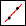(C Line Inf by 2 Points).
2. Choose one of the following:
Straight construction geometry
To create a construction line by two points,
1. Click2 Points in the Construction Line section. The C Line by 2 Points dialog box opens.
2. Click two points on the line, or enter their coordinates in the user input line.
To create a construction line by point and angle,
1. ClickPoint & Angle in the Construction Line section. The C Line Angle dialog box opens.
2. Click the begin point of the line, or enter its coordinates in the user input line.
3. Enter an angle in the user input line, measured from the positive x-axis, or click the second point.
To create an infinite horizontal construction line,
1. Click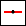Horizontal in the Construction Line section. The C Line Horizontal dialog box opens.
2. Click a point on the line, or enter an offset from y = 0 in the user input line.
To create an infinite vertical construction line,
1. Click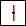Vertical in the Construction Line section. The C Line Vertical dialog box opens.
2. Click a point on the line, or enter an offset from x = 0 in the user input line.
To create an infinite construction line parallel to an existing line,
1. Click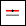Parallel in the Construction Line section. The C Line Parallel to dialog box opens.
2. Click the existing line to draw parallel to.
3. Click a point where you want the construction line to be drawn.
Alternatively,
1. ClickParallel in the Construction Line section. The C Line Parallel to dialog box opens.
2. Enter the required distance between the existing line and the new construction line in the user input line. (You can enter a series of values.)
3. Click the existing line.
4. Click the positive side of the existing line (the side on which the parallel construction line should be drawn).
To create an infinite construction line perpendicular to an existing line,
1. Click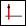Perpendicular in the Construction Line section. The C Line Perpendicular dialog box opens.
2. Click the existing line to draw from perpendicularly.
3. Click a point on the new construction line.
To create a construction line that bisects two points,
You can draw a line which bisects two specified points. It is like drawing a line perpendicular to an imaginary line defined by the two points.
1. ClickBisector in the Construction Line section. The C Line Angle Bisector dialog box opens.
2. Click the two points for the construction line to bisect, or enter their coordinates in the user input line.
Alternatively, you can specify a bisection factor. The range 0 to 1 represents the distance between the first specified point and the second. The bisection factor offsets the bisection line proportional to this distance. 0.5 (midway between the points) is the default bisection.
To draw an infinite construction line using a bisection factor,
1. ClickBisector in the Construction Line section. The C Line Angle Bisector dialog box opens.
2. Enter a bisection factor in the user input line.
3. Click the two points to bisect, or enter their coordinates in the user input line.
To create an infinite construction line by semiangle,
You can draw a line which passes through a specified apex and bisects two specified lines also passing through the apex. The two lines define an angle at the apex, and the construction line is drawn at a semiangle between them.
1. Click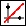Angle Sector in the Construction Line section. The C Line by Semiangle dialog box opens.
2. Click the apex point, or enter its coordinates in the user input line.
3. Specify the semiangle construction line in one of the following ways:
Click two points for the construction line to bisect.
Enter coordinates for the two points in the user input line.
Enter two angles (measured from the positive x-axis) through the apex, defining two lines to bisect.
Alternatively, you can specify a bisection factor. The range 0 to 1 represents the angle between the first specified line through the apex and the second. The bisection factor offsets the semiangle construction line proportional to this angle. 0.5 (midway between the lines) is the default semiangle.
To draw an infinite construction line using a bisection factor,
1. ClickAngle Sector in the Construction Line section. The C Line by Semiangle dialog box opens.
2. Enter a bisection factor in the user input line.
3. Click the apex point, or enter its coordinates in the user input line.
4. Specify the semiangle construction line in one of the following ways:
Click two points for the construction line to bisect.
Enter coordinates for the two points in the user input line.
Enter two angles (measured from the positive x-axis) through the apex, defining two lines to bisect.
To create an infinite construction line tangent to a circular element at a given point or angle,
1. Click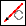Tangent in the Construction Line section. The C Line Tan Point dialog box opens.
2. Specify the approximate point of tangency on the circular element. This can be a circle or an arc.
3. Click a point through which the tangential construction line should be drawn, or enter an angle for the line.
To create an infinite construction line tangent to two circular elements,
1. Click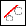Tangent by 2 in the Construction Line section. The C Line Tangent by 2 dialog box opens.
2. Specify the approximate points of tangency on each of two circular elements. These can be circles or arcs.
Creo Elements/Direct Annotation draws a construction line between the two circular elements from points of tangency closest to those specified.
Circular construction geometry
1. Click Geometry and then, in the Draw group, click the arrow next to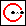(Center & Radius).
2. Choose one of the following:
To create a construction circle by specifying its center and radius,
1. ClickCenter & Radius in the Construction Circle section. The C Circle Cen Radius dialog box opens.
2. Click the center point of the circle, or enter its coordinates in the user input line.
3. Specify the radius of the circle in one of the following ways:
Click a point on the circumference, or enter coordinates in the user input line.
Enter the radius in the user input line.
To create a construction circle concentric to an existing circle or arc,
1. Click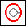Concentric in the Construction Circle section. The C Circle Concentric dialog box opens.
2. Click the existing circle or arc.
3. Specify the offset of the new construction circle in one of the following ways:
Click a point on the circumference, or enter coordinates in the user input line.
Enter the offset of the new circle in the user input line. (A positive value increases the radius, a negative value decreases it.)
To create a construction circle by specifying three points on its circumference,
1. Click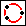3 Points in the Construction Circle section. The C Circle 3 Points dialog box opens.
2. Click three circumference points on the circle, or enter their coordinates in the user input line.
To create a construction circle with a given diameter,
1. Click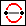Diameter in the Construction Circle section. The C Circle Diameter dialog box opens.
2. Click two diameter end points for the circle, or enter their coordinates in the user input line.
To create a construction circle by two tangents and a point,
You can draw a construction circle with a given radius tangential to two existing elements. The elements of tangency can be lines, arcs or circles.
1. Click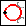Tangent by 2 in the Construction Circle section. The C Circle Tangent by 2 dialog box opens.
2. Click approximate tangent points on two elements.
3. Specify the radius for the new circle in one of the following ways:
Click a point on the circumference, or enter coordinates in the user input line.
Enter the radius in the user input line.
Creo Elements/Direct Annotation calculates the best possible solution.
To create a construction circle by three tangents,
You can draw a construction circle tangential to three existing elements. The elements of tangency can be lines, arcs or circles.
1. Click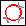Tangent by 3 in the Construction Circle section. The C Circle Tangent by 3 dialog box opens.
2. Click approximate tangent points on three elements.
Creo Elements/Direct Annotation calculates the best possible solution for the circle.
To create a construction circle by tangent and two points,
You can draw a construction circle by specifying a point of tangency with an existing line, circle, or arc, and then two other points on the new circle.
1. Click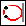Tangent & 2 Points in the Construction Circle section. The C Circle Tan 2 Pnts dialog box opens.
2. Click the existing circle, arc, or line to be tangent to.
3. Click one point on the new circle, or enter its coordinates in the user input line.
4. Specify the circumference of the circle in one of the following ways:
Click a second point on the circle, or enter its coordinates in the user input line.
Enter the radius for the new circle in the user input line.
To create a construction circle by tangents and center,
You can draw a construction circle with a given center tangential to an existing line, arc or circle.
1. ClickTangent & Center in the Construction Circle section. The C Circle Tangent Center dialog box opens.
2. Click an approximate tangent point on an element.
3. Click the new circle's center point, or enter its coordinates in the user input line.
3. Continue drawing construction geometry or click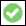to complete the operation.